# Magnetic field associated with a current  (Page 2/2)

 Page 2 / 2

## Case study : the right hand rule

Use the Right Hand Rule to draw in the directions of the magnetic fields for the following conductorswith the currents flowing in the directions shown by the arrows. The first problem has been completed for you.

 1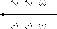2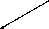3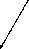45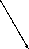6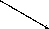78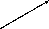9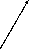1011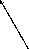12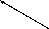## Experiment : magnetic field around a current carrying conductor

Apparatus:

1. one 9V battery with holder
2. two hookup wires with alligator clips
3. compass
4. stop watch

Method:

1. Connect your wires to the battery leaving one end of each wire unconnected so that the circuit is not closed.
2. One student should be in charge of limiting the current flow to 10 seconds. This is to preserve battery life as well as to prevent overheating of the wires and battery contacts.
3. Place the compass close to the wire.
4. Close the circuit and observe what happens to the compass.
5. Reverse the polarity of the battery and close the circuit. Observe what happens to the compass.

Conclusions:

Use your observations to answer the following questions:

1. Does a current flowing in a wire generate a magnetic field?
2. Is the magnetic field present when the current is not flowing?
3. Does the direction of the magnetic field produced by a current in a wire depend on the direction of the current flow?
4. How does the direction of the current affect the magnetic field?

## Case study : magnetic field around a loop of conductor

Consider two loops made from a conducting material, which carry currents (in opposite directions) and are placed in the planeof the page. By using the Right Hand Rule, draw what you think the magnetic field would look like at different points around each of the twoloops. Loop 1 has the current flowing in a counter-clockwise direction, while loop 2 has the current flowing in a clockwisedirection.

If you make a loop of current carrying conductor, then the direction of the magnetic field is obtained by applying the RightHand Rule to different points in the loop.

If we now add another loop with the current in the same direction, then the magnetic field around each loop can be added together to create a stronger magnetic field. A coil of many such loops is called a solenoid . The magnetic field pattern around a solenoid is similar to the magnetic field pattern around the bar magnet that you studied in Grade 10, which had a definite north and south pole.

## Electromagnets

An electromagnet is a piece of wire intended to generate a magnetic field with the passage of electric current through it.Though all current-carrying conductors produce magnetic fields, an electromagnet is usually constructed in such a way as to maximizethe strength of the magnetic field it produces for a special purpose. Electromagnets are commonly used in research,industry, medical, and consumer products. An example of a commonly used electromagnet is in security doors, e.g. on shop doors which open automatically.

As an electrically-controllable magnet, electromagnets form part of a wide variety of "electromechanical" devices: machines that produce a mechanical force or motion through electricalpower. Perhaps the most obvious example of such a machine is the electric motor which will be described in detail in Grade 12. Other examples of the use of electromagnets are electric bells, relays, loudspeakers and scrapyard cranes.

## Experiment : electromagnets

Aim:

A magnetic field is created when an electric current flows through a wire. A single wire does not produce a strong magnetic field,but a wire coiled around an iron core does. We will investigate this behaviour.

Apparatus:

1. a battery and holder
2. a length of wire
3. a compass
4. a few nails

Method:

1. If you have not done the previous experiment in this chapter do it now.
2. Bend the wire into a series of coils before attaching it to the battery. Observe what happens to the deflection of the needle on the compass. Has the deflection of the compass grown stronger?
3. Repeat the experiment by changing the number and size of the coils in the wire. Observe what happens to the deflection on the compass.
4. Coil the wire around an iron nail and then attach the coil to the battery. Observe what happens to the deflection of the compass needle.

Conclusions:

1. Does the number of coils affect the strength of the magnetic field?
2. Does the iron nail increase or decrease the strength of the magnetic field?

## Magnetic fields

1. Give evidence for the existence of a magnetic field near a current carrying wire.
2. Describe how you would use your right hand to determine the direction of a magnetic field around a current carrying conductor.
3. Use the Right Hand Rule to determine the direction of the magnetic field for the following situations:
4. Use the Right Hand Rule to find the direction of the magnetic fields at each of the points labelled A - H in the following diagrams.

#### Questions & Answers

Why do vectors have arrows?
vector quantity has both direction and magnitude so arrows illustrate the direction of the vector
Mhleli
how to calculate net electric field at a point
what are controlled variables
Independent variables in an experiment which influence the outcome results
Boss
Such as temperature when dealing with ohms law
Boss
variables whose amount is determined by the person doing the experiment
Clemencia
how to calculate exodation number
Calculate relative atomic mass
what is chemical bonding?
Chemical Bonding is a mutual attraction between two atoms resulting from the simultaneous attraction between their nuclei and the outer elections
Sydney
How would you go about finding the resistance of an unknown resistor using only power supply, a voltmeter and a known resistance
Lebona
who is the scientist who discovered electromagnetism
what happens to the galvanometer when the switch is closed ?
Zivele
how do we identify an oxidising or reducing agent in a reaction?
Zivele
hey
Banele
what is electricity
Formula for concentration
c=m/MV
Zipho
if given number of moles and volume , can use c=n/V
sibahle
Chemistry term three topic is stressing me out, I really need a huge help
Ziyanda
on what
Olebogeng
during a snooker competition ,a 200g ball A m moving with velocity va collide head on with a identical ball B that was at rest.A after the collision ball A remains at rest wile ball B moves on with a velocity of 4m/s? With what speed was ball a moving before the collision
a vector can be resolved into a horizontal component only
how to calculate normal force
how to calculate wavelength
Lizoh
Hello. How does a real gas behave under low temperature and high pressure?
does a vector quantity include force and distance?
yes
Itumeleng
what's the difference between a vector and a scalar?
Keaobaka
vector is the physical quantity with magnitude and direction Scalar is the Physical quantity with magnitude only
Bobo
Newton's second law of motion
Newton second law motin
Shife
Newton's second law of motion: When a resultant/net force acts on an object, the object will accelerate in the direction of the force at an acceleration directly proportional to the force and inversely proportional to the mass of the object.
Bobo
newtons third law of motion
Ayakha
when object A exert a force on object B object B SIMULTANEOUS exert a force of equal magnitude on object A
TshepisoBy Anindyo MukhopadhyayBy Abishek DevarajByBy